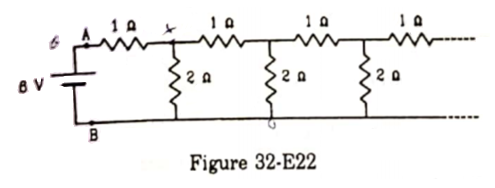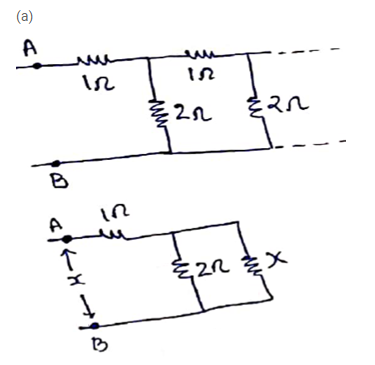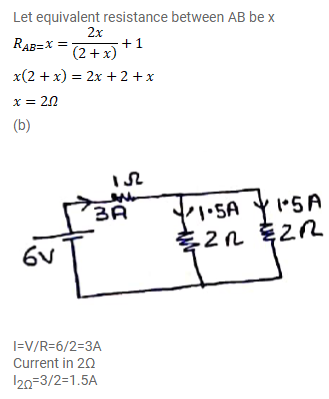# An infinite ladder is constructed with 1Ω and 2Ω resistors as

Question:

An infinite ladder is constructed with $1 \Omega$ and $2 \Omega$ resistors as shown in figure (32-E22).

(a) Find the effective resistance between the points $A$ and $B$.

(b) Find the current that passes through the $2 \Omega$ resistor nearest to the battery.Solution: